Orientation Density Functions edit page

This example demonstrates the most important MTEX tools for analysing Pole Figure Data.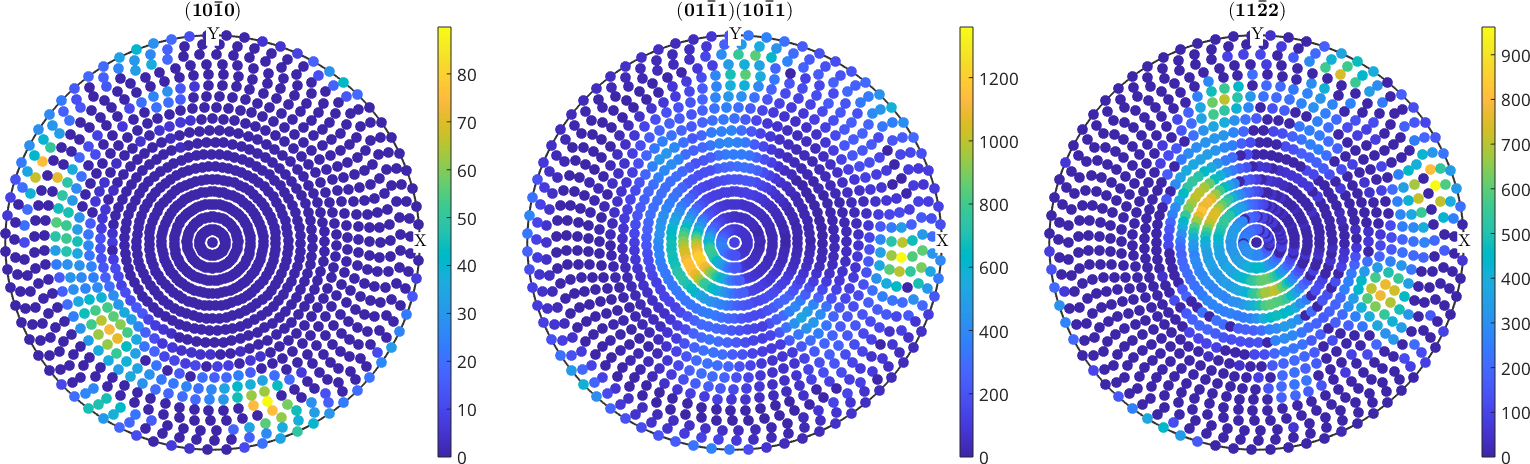## Extract information from imported pole figure data

Get raw data

Data stored in a PoleFigure variable can be extracted by

Basic Statistics

There are also some basic statics on pole figure intensities

## Manipulate pole figure data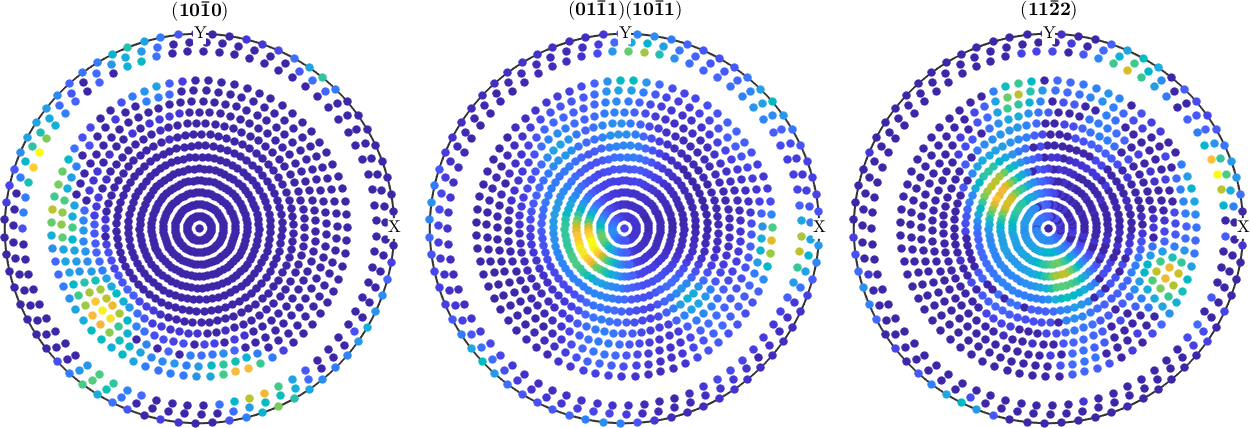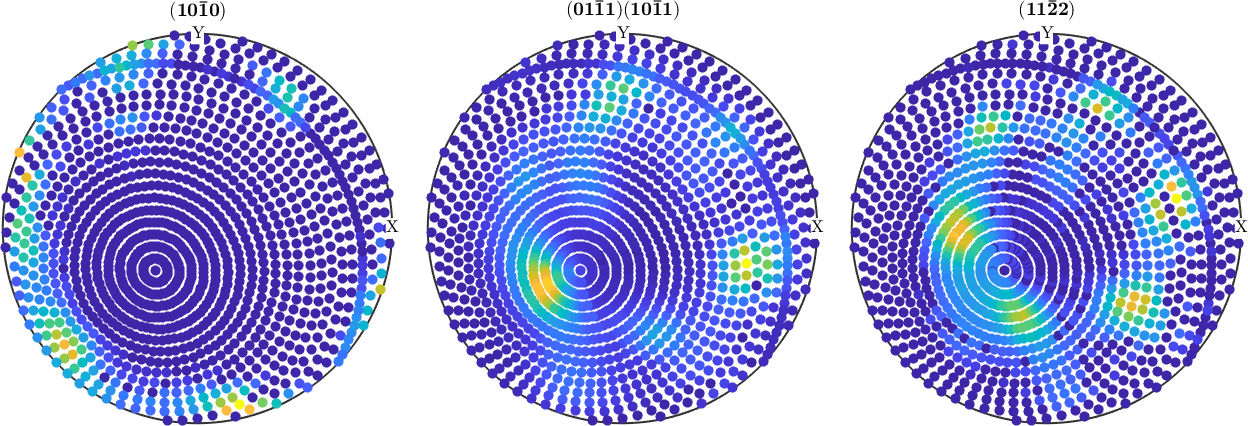## PDF - to - ODF Reconstruction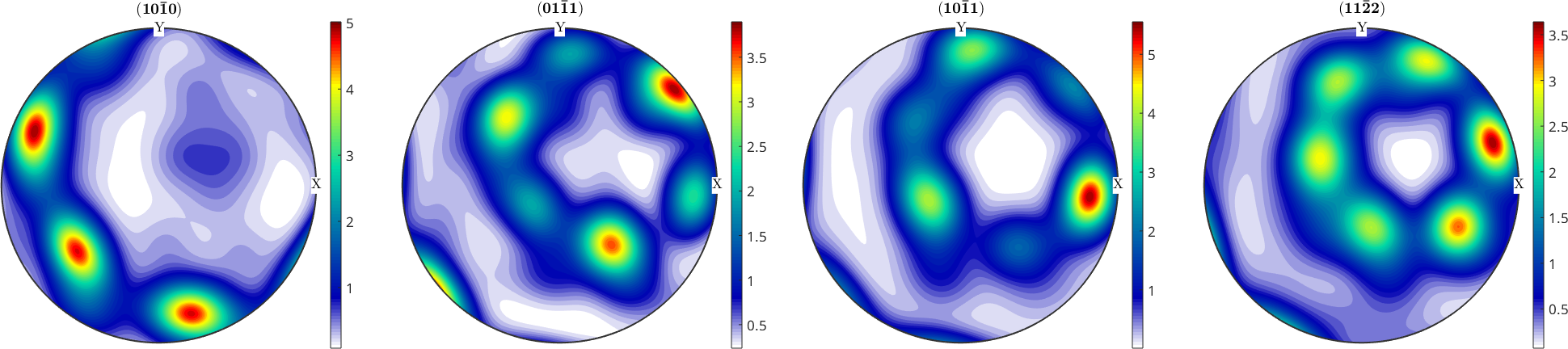define crystal directions

simulate pole figure data

estimate an ODF with ghost correction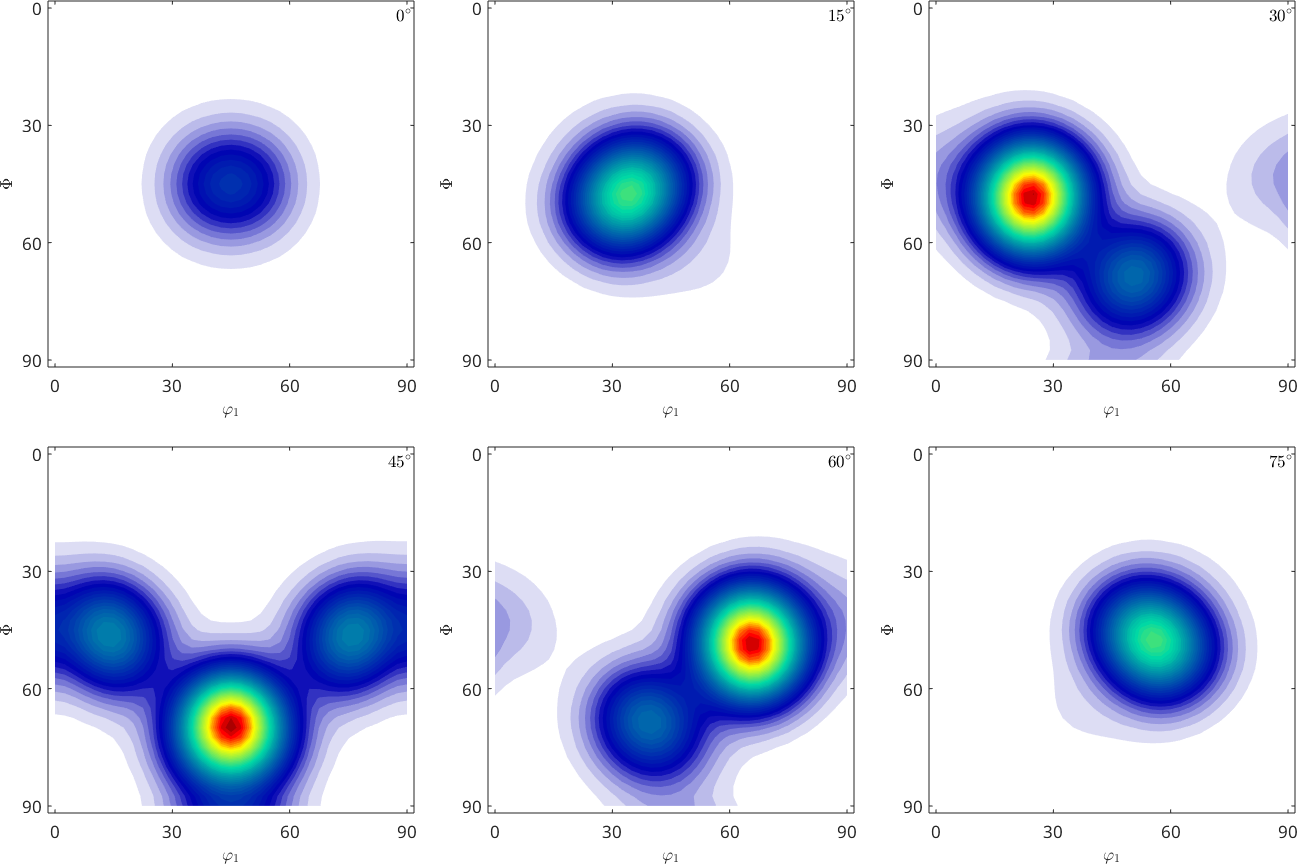without ghost correction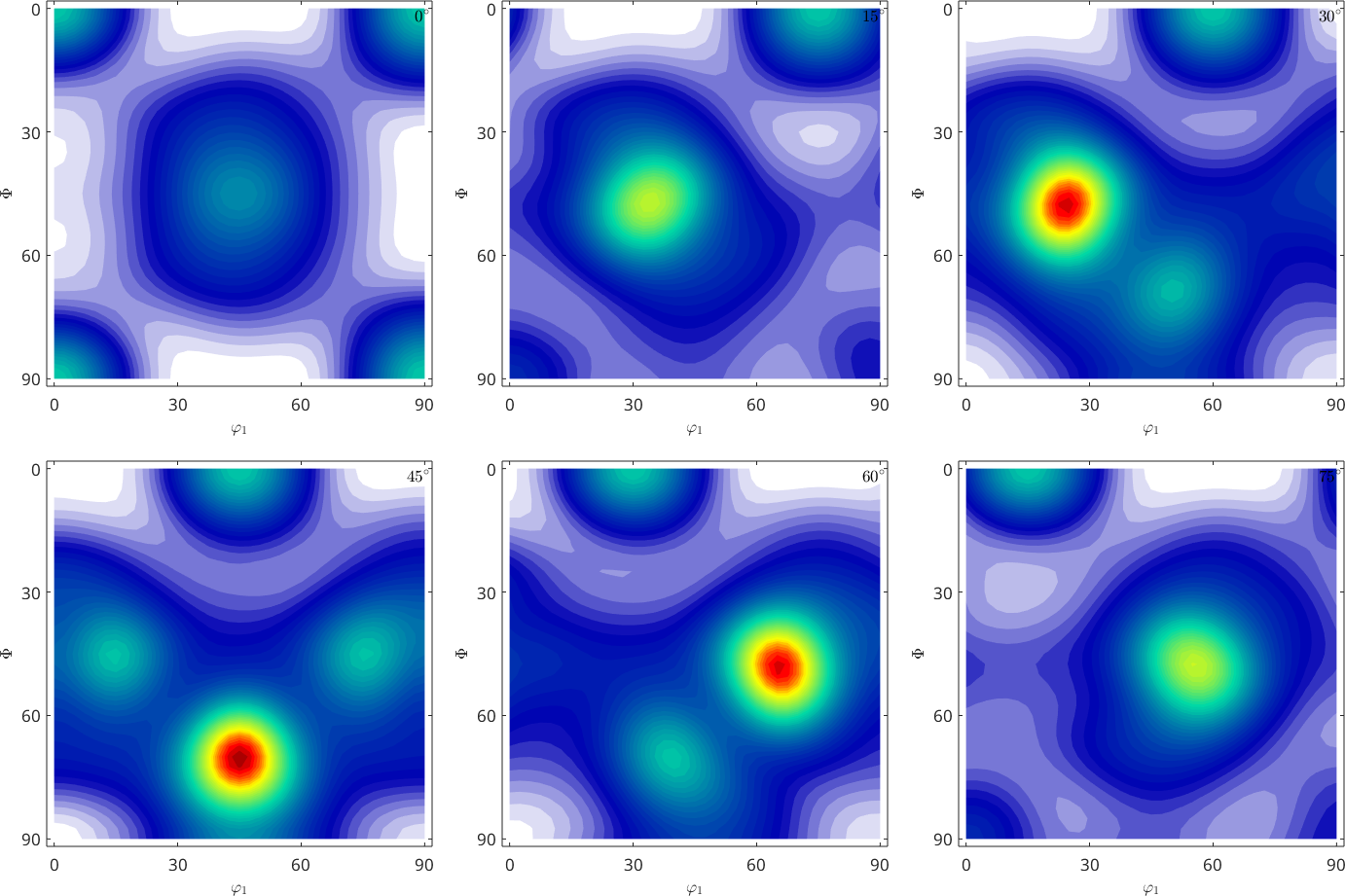## Error Analysis

Difference plot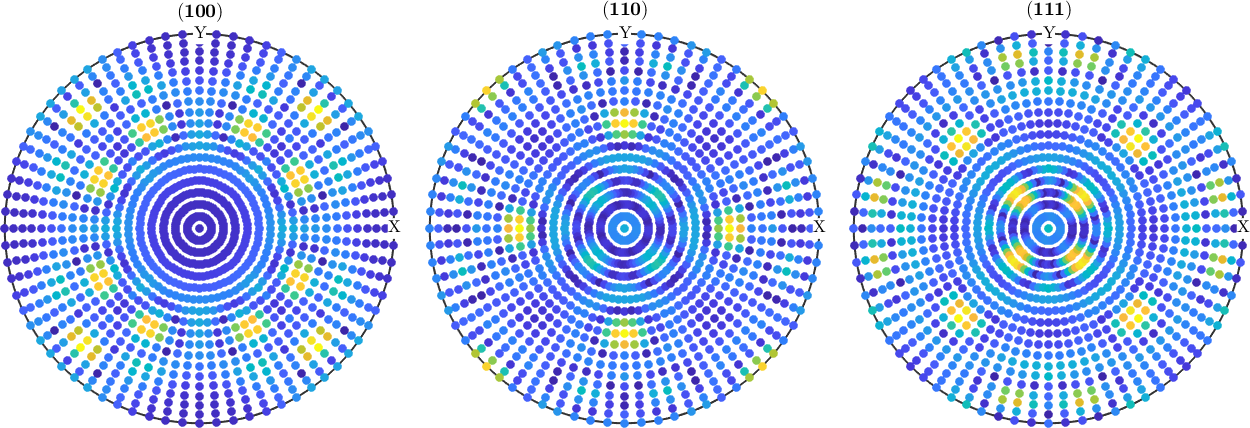ODF error

## Exercises

3)

a) Load the pole figure data of a quartz specimen from: data/dubna!

b) Inspect the raw data. Are there noticeable problems?

c) Compute an ODF from the pole figure data.

d) Plot some pole figures of that ODF and compare them to the measured pole figures.

e) Compute the RP errors for each pole figure.

f) Plot the difference between the raw data and the calculated pole figures. What do you observe?

g) Remove the erroneous values from the pole figure data and repeat the ODF calculation. How do the RP error change?

h) Vary the number of pole figures used for the ODF calculation. What is the minimum set of pole figures needed to obtain a meaningful ODF?